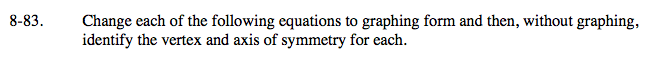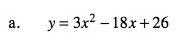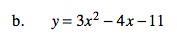### Home > A2C > Chapter 8 > Lesson 8.1.5 > Problem8-83

8-83.Complete the square to get the equation in graphing form.

The axis of symmetry is the same as the x-coordinate of the vertex.vertex: (3, −1)
axis of symmetry: x = 3